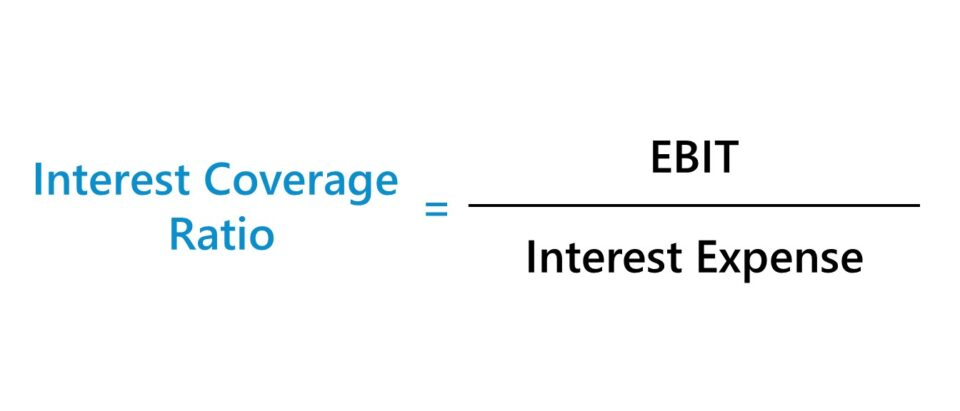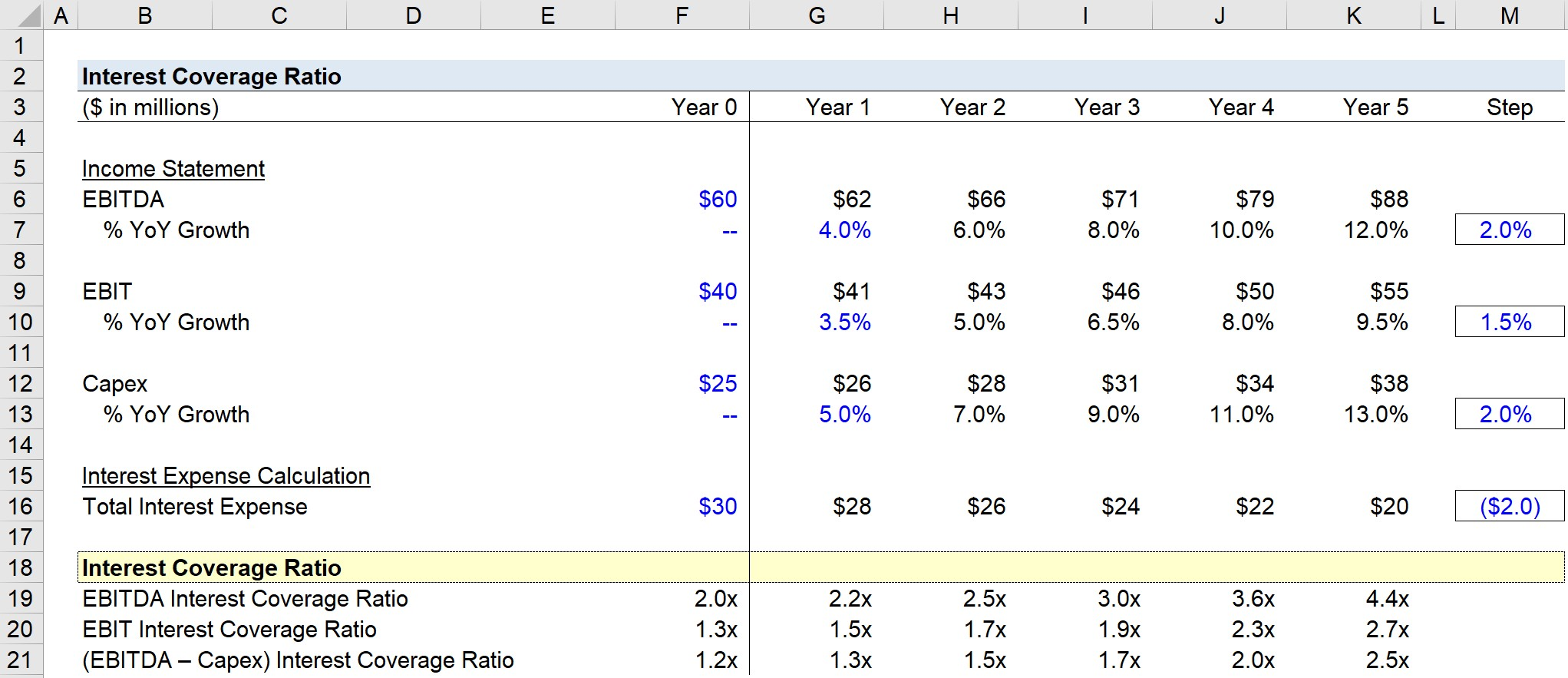Welcome to Wall Street Prep! Use code at checkout for 15% off.# Interest Coverage Ratio (ICR)

Guide to Understanding the Interest Coverage Ratio (ICR)## How to Calculate Interest Coverage Ratio?

The interest coverage ratio (ICR) measures the ability of a company to meet scheduled interest obligations coming due on time.

Besides the mandatory repayment of the original debt principal by the date of maturity, the borrower must also service its interest expense payments on schedule to avoid defaulting.

The more debt principal that a company has on its balance sheet, the more interest expense the company will owe to its lenders — all else being equal.

In contrast to leverage ratios, coverage ratios compare a cash flow metric that captures the company’s operating cash flow in the numerator to the amount of interest expense on the denominator.

Operating Cash Flow Metrics:

Of the four metrics, EBITDA tends to output the highest value for an interest coverage ratio since D&A is added back, while “EBITDA – Capex” is the most conservative.

## Interest Coverage Ratio Formula

The formula to calculate the interest coverage ratio involves dividing a company’s operating cash flow metric – as mentioned earlier – by the interest expense burden.

Interest Coverage Ratio (ICR) = EBIT ÷ Interest Expense

The EBIT interest coverage ratio tends to be the most commonly used because it represents the conservative, “middle ground.”

Usually, when practitioners mention the “interest coverage ratio”, it is reasonable to assume they are referring to EBIT.

## Quick Interest Coverage Ratio Calculation Example

For instance, if the EBIT of a company is \$100 million while the amount of annual interest expense due is \$20 million, the interest coverage ratio is 5.0x.

• EBIT Coverage Ratio = \$100m ÷ \$20m = 5.0x

The EBIT of the company can service the \$20m in interest expense five times, which means the company’s operating earnings can pay its current interest payment for five “turns.”

But if the EBIT coverage ratio were hypothetically much lower, let’s say only 1.0x, for example, just a slight drop-off in performance for the company could cause a default due to a missed interest expense payment.

## What is a Good Interest Coverage Ratio?

For purposes related to lending to a potential borrower and/or providing other forms of capital, interest coverage ratios can be helpful in understanding whether the company’s cash flows are sufficient to pay off the required interest payments on its debt.

• High Leverage Ratios→ Higher leverage ratios equate to more financial risk, meaning the borrower’s probability of defaulting on its required debt payments becomes more of a concern.
• High Interest Coverage Ratios (ICR)→ For coverage ratios, however, the lower the number, the riskier the credit health of the borrower – which is the opposite of leverage ratios.

Therefore, the higher the number of “turns” for an interest coverage ratio, the more coverage (and reduced risk), because there is more “cushion” in case the company underperforms.

## What are the Different Types of Interest Coverage Ratios?

 Coverage Ratio Formula Purpose EBITDA Interest Coverage Ratio EBITDA Interest Coverage Ratio = EBITDA ÷ Interest Expense The EBITDA coverage ratio measures the number of times EBITDA can service the interest expense coming due. EBIT Interest Coverage Ratio EBIT Interest Coverage Ratio = EBIT ÷ Interest Expense The EBIT coverage ratio measures the number of times EBIT can service the interest expense coming due. EBITDA Less Capex Interest Coverage Ratio EBITDA Less Capex Interest Coverage Ratio = (EBITDA – Capex) ÷ Interest Expense The EBITDA Less Capex coverage ratio measures the number of times that EBITDA, once Capex is deducted, can service the interest expense coming due Fixed Charge Coverage Ratio (FCCR) Fixed Charge Coverage Ratio (FCCR) = (EBITDA – Capex) ÷ (Interest Expense + Current Portion of Long-Term Debt) The fixed charge coverage ratio (FCCR) measures a company’s ability to service all required, short-term financial obligations – can often adjust for rent expense as well

## What is the Difference Between Coverage Ratio vs. Leverage Ratio?

Another method to measure risk is leverage ratios, which determine how much debt comprises the entire capital structure. Here, the amount of debt carried by a company is compared to either:

## Interest Coverage Ratio Calculator

We’ll now move to a modeling exercise, which you can access by filling out the form below.Submitting...

## 1. Operating Assumptions

Suppose a company had the following select income statement financial data in Year 0.

• EBITDA = \$60 million
• EBIT = \$40 million
• Capex = \$25 million
• Total Interest Expense = \$30 million

From Year 1 onward, we’ll use a step function that assumes each line item will grow by the following:

• EBITDA: 4.0% Growth Rate in Year 1 and Increase of +2.0% / Year
• EBIT: 3.5% Growth Rate in Year 1 and Increase of +1.5% / Year
• Capex: 5.0% Growth Rate in Year 1 and Increase of +2.0% / Year
• Total Interest Expense: Decline by –\$2m / Year

## 2. Financial Forecast

By the end of Year 5, EBITDA is growing at 12.0% year-over-year (YoY), EBIT is growing by 9.5%, and Capex is growing at 13.0%, which shows how the company’s operations are growing.

However, the pace of the required reinvestments (i.e. Capex) to fund the growth is also rapidly increasing in line with the EBITDA growth.

In contrast, the company’s total interest expense is declining from \$30m in Year 0 to \$20m by the end of Year 5, suggesting the company’s debt principal is declining, which directly leads to lower interest expense since interest is a function of the amount of the outstanding debt principal.

## 3. Interest Coverage Ratio Calculation Example

Once all the forecasted years have been filled out, we can now calculate the three key variations of the interest coverage ratio.

For each variation, we’ll divide the appropriate cash flow metric by the total interest expense amount due in that particular year.

From Year 0 to Year 5, the interest coverage ratio in each period undergoes the following shifts:

• EBITDA Coverage Ratio: 2.0x → 4.4x
• EBIT Coverage Ratio: 1.3x → 2.7x
• (EBITDA – Capex) Coverage Ratio: 1.2x → 2.5x

Given the outpacing of EBITDA vs. EBIT and the growth of Capex being on par with the growth of EBITDA, the EBITDA variation of the calculated interest coverage ratio is the highest, whereas the (EBITDA – Capex) variation is the lowest of the three types, with the EBIT variation coming in the middle.### The Wharton Online and Wall Street Prep Private Equity Certificate Program

Level up your career with the world's most recognized private equity investing program. Enrollment is open for the Jan. 29 - Mar. 25 cohort.